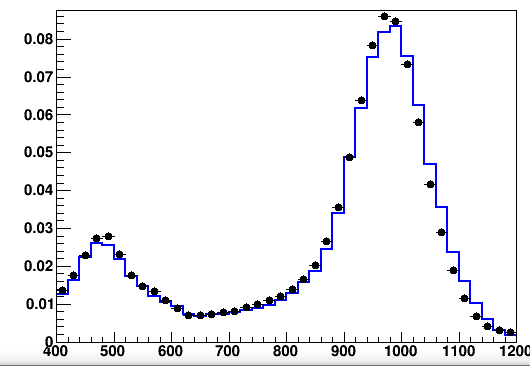# [question] GetBinContentHello ROOTers…

``````TCanvas *c3 = new TCanvas("c3", "residual", 1200, 900);
TH1F *h1 = new TH1F("h1", "residual", 40, 400, 1200);
hQtot_Bi->SetNormFactor(1);
hQtot_Bi_d->SetNormFactor(1);
for(Int_t i=1; i<=1200; i++) {
if (i<400) continue;
Double_t res = ((hQtot_Bi_d->GetBinContent(i) - hQtot_Bi->GetBinContent(i))/hQtot_Bi->GetBinContent(i))*100;
h1->SetBinContent(i, res);
cout << "i = " << i << ", res = " << res << endl;
}
h1->SetLineColor(kBlack);
h1->SetLineWidth(2);
h1->SetStats(0);
h1->Draw();
``````

I want to get ‘res’ related to hQtot_Bi(blue line) and hQtot_Bi_d(black dots) and plot them. I am trying to plot through ‘res’ of the code. However, when I output the res value, all the same values came out. How can it be solved?

ex) i = 400, res = -94.5654 … i = 1200, res = -94.5654

`for(Int_t i = 1; i <= h1->GetNbinsX(); i++) {`

BTW. See “Convention for numbering bins” in: TH1

Oh… I got it.
Thank you so much!!